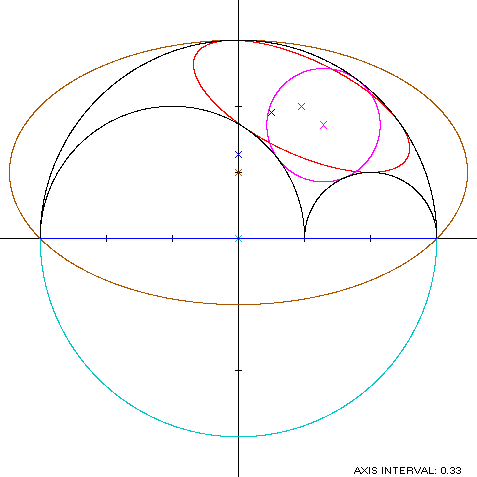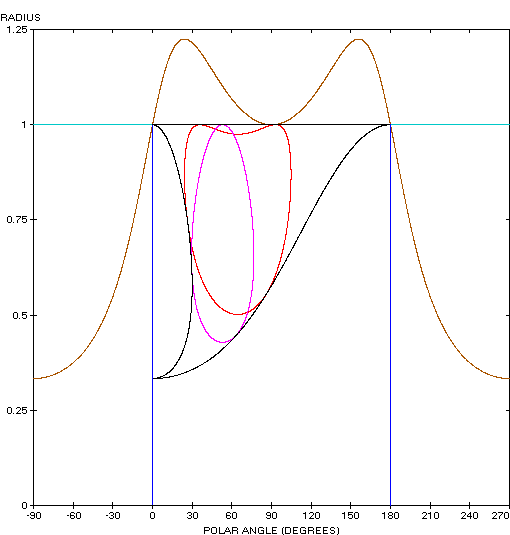Arbelos
ARBELOS

Balmoral Software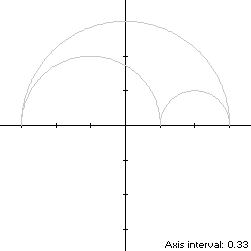Consider the arbelos S with left radius r and right radius 1 - r, 0 < r < 1, and therefore with enclosing radius 1, oriented so that the center of the enclosing semicircle is at the origin. Then the centers of the left and right semicircles are (r - 1,0) and (r,0) respectively. S is asymmetric if r ≠ 1/2.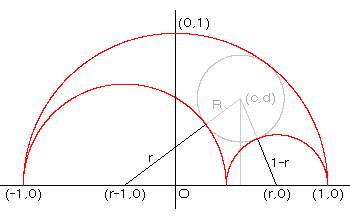So that S is traversed in a counterclockwise direction starting from its right edge (1,0) at t = 0, its parametric equations can be written: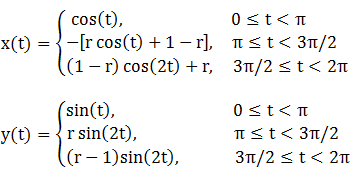### Metrics

The perimeter of the arbelos is the sum of the semicircumferences of circles of radius 1, r and 1 - r, or 2π, the perimeter of a unit circle (the answer from WolframAlpha is in error by a factor of 2). Its area is that of a unit semicircle, less the areas of the two smaller semicircles, or r(1 - r)π, which ranges from 0 to π/4.

Using the formula for the centroid of a semicircle, the arbelos metrics can be summarized as follows:

 Area Centroid Product Region Unit semicircle π/2 (0,4/(3π)) (0,2/3) Left semicircle r2π/2 (r - 1,4r/(3π)) (r2(r - 1)π/2,2r3/3) Right semicircle (1 - r)2π/2 (r,4(1 - r)/(3π)) (r(r - 1)2π/2,2(1 - r)3/3) Arbelos (by subtraction) r(1 - r)π (r(1 - r)(2r - 1)π/2,2r(1 - r))
The centroid of the arbelos is the weighted average
(r(1 - r)(2r - 1)π/2,2r(1 - r))/[r(1 - r)π] = (r - 1/2,2/π)
Since the arbelos has three cusps, it is non-convex by the multiple local extrema test. The width x height of its bounding rectangle is that of the unit semicircle, 2 x 1.

### Convex Hull

The convex hull of the arbelos is created simply by connecting its three cusps with a horizontal line segment of length 2, as shown in blue in the left diagram below. The perimeter of the convex hull is half the circumference of a unit circle plus the line segment, or π + 2, which is about 18% shorter than the perimeter of the arbelos. The area of the convex hull is that of a unit semicircle, or π/2, which is always at least twice the area of the arbelos itself. The centroid of the convex hull is that of a unit semicircle centered at the origin, as shown above: (0,4/(3π)). When r is greater than 8/(9π2) + 1/2 = 0.59, the centroid of the convex hull is outside of the arbelos.

### Circumellipse

The extreme points of the arbelos create a y-symmetric isosceles triangle with vertices at (0,1) and (±1,0). Using h = 1 and w = 2 in Lemma TE, a candidate for the circumellipse has parameters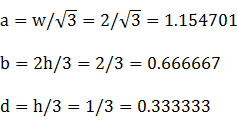The parametric equations of the convex hull of S are: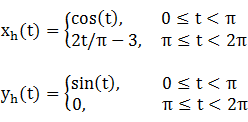For verification,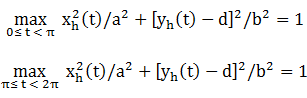### Circumcircle

Since the arbelos is a portion of a unit circle centered at the origin, its circumcircle is that circle.

### Incircle

Denote the radius and center of the largest circle inscribed in the arbelos as R and (c,d), as shown in the diagram above. Since the points of tangency of the incircle with the left and right semicircles lie along lines connecting their centers, we have
[c - (r - 1)]2 + (d - 0)2 = (r + R)2

(c - r)2 + (d - 0)2 = (1 - r + R)2

By "Surprise No. 7" in , a vertical line from (c,d) to the horizontal base of the arbelos is bisected by the lower arc of the incircle; or equivalently,
d = 2R
Solving the preceding three equations simultaneously for R, c and d yields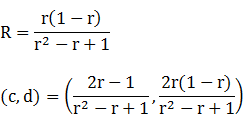Specific Example

In the remainder of this paper, we use r = 2/3 as illustrated in the diagram above. Then the metrics of the incircle are:

R = 2/7

(c,d) = (3/7,4/7)

and the centroid of the arbelos is (1/6,2/π).

### Inellipse

Since it's evident that an optimal inscribed ellipse will be tilted downwards toward the smallest semicircle, we can formulate an equivalent problem by rotating the arbelos itself by a counterclockwise angle -φ to orient its inellipse in a rectilinear position. This ellipse will be tangent to each of the smaller semicircles and twice tangent to the enclosing semicircle, and is y-symmetric. A numerical search can be used to determine the optimal tilted inellipse:
φ = -25.514°

a = 0.592932

b = 0.236724

c = 0.318006

d = 0.666294

Area = 0.440957

### Summary Table

 Perimeter Area Centroid Figure Parameters Incircle R = 2/7 1.795196 0.256457 (0.428571,0.571429) Inellipse a = 0.592932b = 0.236724 2.728001 0.440957 (0.318006,0.666294) Arbelos Width: 2Height: 1 6.283185 0.698132 (0.166667,0.636620) Convex hull 5.141593 1.570796 (0,0.424413) Circumellipse5.825171 2.418401 (0,0.333333) Circumcircle R = 1 6.283185 3.141593